Question

# The equilibrium constant for the chemical equation N2(g)+3H2(g)↽−−⇀2NH3(g) is ?p=0.0752 at 221 ∘C. Calculate the value...

The equilibrium constant for the chemical equation N2(g)+3H2(g)↽−−⇀2NH3(g) is ?p=0.0752 at 221 ∘C. Calculate the value of ?c for the reaction at 221 ∘C.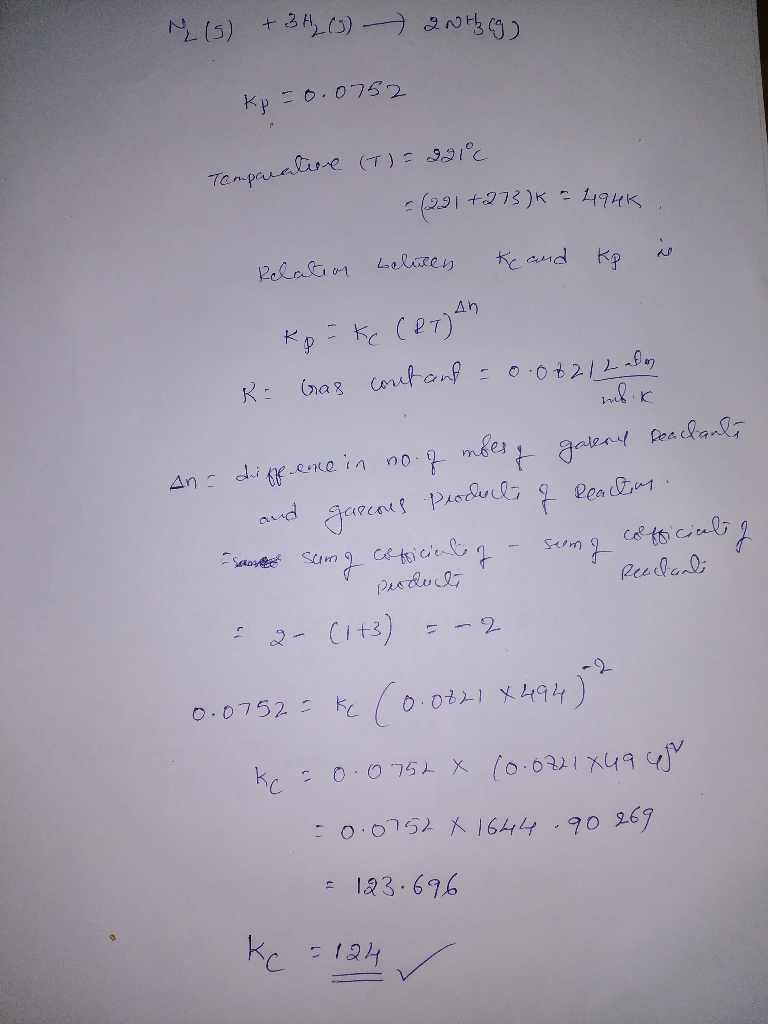#### Earn Coins

Coins can be redeemed for fabulous gifts.

Similar Homework Help Questions
• ### The equilibrium constant for the chemical equation N2(g)+3H2(g)−⇀↽−2NH3(g) is Kp=0.111 at 231 ∘C. Calculate the value...

The equilibrium constant for the chemical equation N2(g)+3H2(g)−⇀↽−2NH3(g) is Kp=0.111 at 231 ∘C. Calculate the value of Kc for the reaction at 231 ∘C.

• ### The equilibrium constant for the chemical equation N,(g) + 3H2(8) - 2NH3(g) is Kp = 0.0881...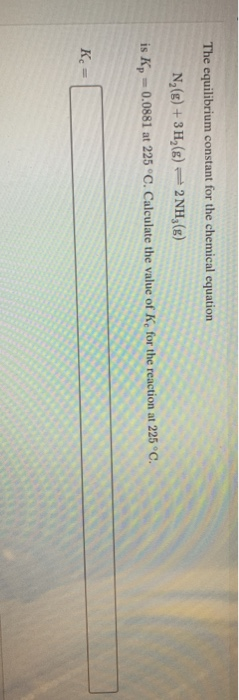The equilibrium constant for the chemical equation N,(g) + 3H2(8) - 2NH3(g) is Kp = 0.0881 at 225 °C. Calculate the value of K, for the reaction at 225°C. Kc =

• ### The equilibrium constant for the chemical equation N2(g) 3 H2(g)2NH3 (g) is Kp = 0.0146 at...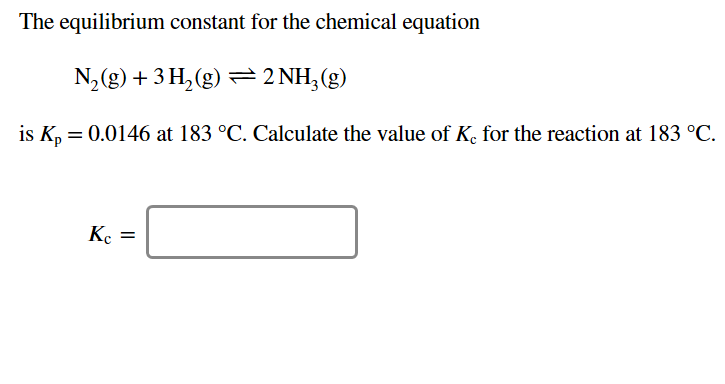The equilibrium constant for the chemical equation N2(g) 3 H2(g)2NH3 (g) is Kp = 0.0146 at 183 oC. Calculate the value of Kc for the reaction at 183 °C.

• ### The equilibrium constant for the chemical equation N2 g) +3H 2(g) 근 2NH3(g) is Kp =...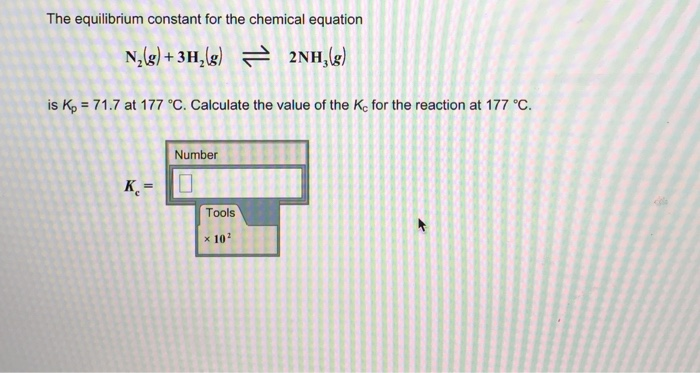The equilibrium constant for the chemical equation N2 g) +3H 2(g) 근 2NH3(g) is Kp = 71.7 at 177 °C. Calculate the value of the Ke for the reaction at 177 °C. Number K,= Tools x 102

• ### Consider the chemical reaction 2NH3(g) ó N2(g) + 3H2(g). The equilibrium is to be established in...

Consider the chemical reaction 2NH3(g) ó N2(g) + 3H2(g). The equilibrium is to be established in a 50.0 L container at 1,000 K, where Kc = 4.0 × 10-2. Initially, 6.10 x 105 moles of NH3(g) are present. Calculate the amount of H2 presentat equilibrium. [H2] =_____

• ### For the reaction N2(g)+3H2(g)⇌2NH3(g) what is the value of Kc at 500 ∘C if the equilibrium...

For the reaction N2(g)+3H2(g)⇌2NH3(g) what is the value of Kc at 500 ∘C if the equilibrium concentrations are as follows: [H2] = 0.45 M , [N2] = 0.50 M , and [NH3] = 2.0 M ?

• ### The equilibrium constant for the chemical equation N,(8)+ 3H2() – 2NH3(8) is k, = 0.0301 at...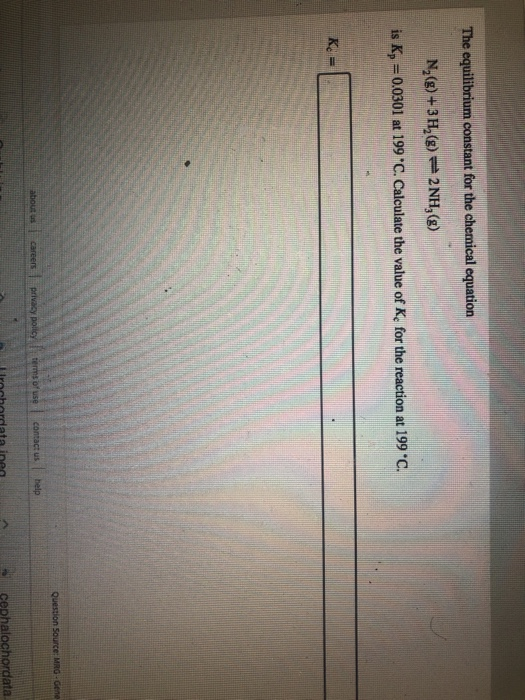The equilibrium constant for the chemical equation N,(8)+ 3H2() – 2NH3(8) is k, = 0.0301 at 199 *C. Calculate the value of K, for the reaction at 199 C. Question Source: MRG - Gene about Careers Privacy policy contact us Prochordata ines cephalochordata

• ### For the reaction N2(g)+3H2(g)⇌2NH3(g) what is the value of Kc at 500 ∘C if the equilibrium...

For the reaction N2(g)+3H2(g)⇌2NH3(g) what is the value of Kc at 500 ∘C if the equilibrium concentrations are as follows: [H2] = 0.35 M , [N2] = 0.40 M , and [NH3] = 1.8 M ?how to solve answer?

• ### The equilibrium constant, K, for the following reaction is 6.30 at 723K. 2NH3(g) N2(g) + 3H2(g)...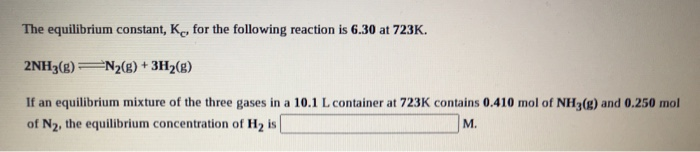The equilibrium constant, K, for the following reaction is 6.30 at 723K. 2NH3(g) N2(g) + 3H2(g) If an equilibrium mixture of the three gases in a 10.1 L container at 723K contains 0.410 mol of NH3(g) and 0.250 mol of N2, the equilibrium concentration of Hy is M.

• ### The equilibrium constant, Kc, for the following reaction is 6.30 at 723K. 2NH3(g) N2(g) + 3H2(g)...

The equilibrium constant, Kc, for the following reaction is 6.30 at 723K. 2NH3(g) N2(g) + 3H2(g) If an equilibrium mixture of the three gases in a 15.7 L container at 723K contains 0.284 mol of NH3(g) and 0.437 mol of N2, the equilibrium concentration of H2 is__________ M.